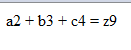The HTML syntax of HTML5 allows MathML (Mathematical Markup Language) element to be used inside a document using [itex] tag. It aims at integrating mathematical formulae into World Wide Web pages and other document. MathML deals not only with the presentation but also the meaning of formula components (the latter part of MathML is known as “Content MathML”). Because the meaning of the equation is preserved separate from the presentation, how the content is communicated can be left up to the user.

For valid XML documents as per the W3C specifications, a MathML file must have a <! DOCTYPE> declaration:

`  <?xml version="1.0" encoding="UTF-8"?>  <!DOCTYPE math PUBLIC "-//W3C//DTD MathML 2.0//EN"           "http://www.w3.org/Math/DTD/mathml2/mathml2.dtd">`

Thus, the expression ax2+bx+c could be represented in a valid MathML file as:

` <?xml version="1.0" encoding="UTF-8"?>  <!DOCTYPE math PUBLIC "-//W3C//DTD MathML 2.0//EN"           "http://www.w3.org/Math/DTD/mathml2/mathml2.dtd">  <math xmlns="http://www.w3.org/1998/Math/MathML">    <mrow>      <mi>a</mi>       <mo>&#x2062;<!-- &InvisibleTimes; --></mo>      <msup>         <mi>x</mi>         <mn>2</mn>      </msup>      <mo>+</mo>      <mi>b</mi>       <mo>&#x2062;<!-- &InvisibleTimes; --></mo>      <mi>x</mi>      <mo>+</mo>      <mi>c</mi>    </mrow>  [/itex]`

This example will display a2+b3+c4=z9 could be represented in a valid MathML file as:

`<head>     <title>MathML Examples</title></head><body>     <math><!--using math tag-->   <mrow>   <msup>   <mi>a</mi><mn>2</mn>   <mo>+</mo>   </msup>   <msup>   <mi>b</mi><mi>3</mi>   <mo>+</mo>   </msup>   <msup>   <mi>c</mi><mi>4</mi>   <mo>=</mo>   </msup>   <msup><mi>z</mi><mi>9</mi></msup>   </mrow>   </math></body>`

This would produce following result as shown below:Modified On Sep-07-2019 12:12:24 AM